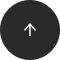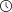- 8 minutes read

# High Performance Statistical Queries –Skewness and Kurtosis

In descriptive statistics, the first four population moments include center, spread, skewness, and kurtosis or peakedness of a distribution. In this article, I am explaining the third and fourth population moments, the skewness and the kurtosis, and how to calculate them.

Mean uses the values on the first degree in the calculation; therefore, it is the first population moment. Standard deviation uses the squared values and is therefore the second population moment. Skewness is the third, and kurtosis is the fourth population moment. All together, they give you a very good estimation of the population distribution.

Before dealing with skewness and kurtosis, let me introduce the normal and standard-normal distributions.

### Normal and Standard Normal Distribution

Normal distributions are a family of distributions that have the same general shape. Normal distributions are symmetric, with scores more concentrated in the middle than in the tails. Normal distributions are described as bell shaped. The bell curve is also called a Gaussian curve, in honor of Karl Friedrich Gauss.

The height of a normal distribution is specified mathematically with two parameters: the mean (µ) and the standard deviation (σ). Constants in the formula are π (3.14159) and e (the base of natural logarithms = 2.718282). The formula for the normal distribution is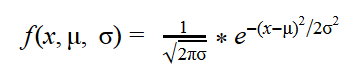Normal distributions are extremely important in statistics and are often used in the natural and social sciences for real-valued random variables whose distributions are not known. Simply said, if you do not know the distribution of a continuous variable in advance, you assume that it follows the normal distribution.

The standard normal distribution (Z distribution) is a normal distribution with a mean of 0 and a standard deviation of 1. You can easily calculate the z values of the standard normal distribution by normalizing the x values of the normal distribution: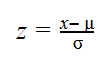The following picture shows the normal distribution.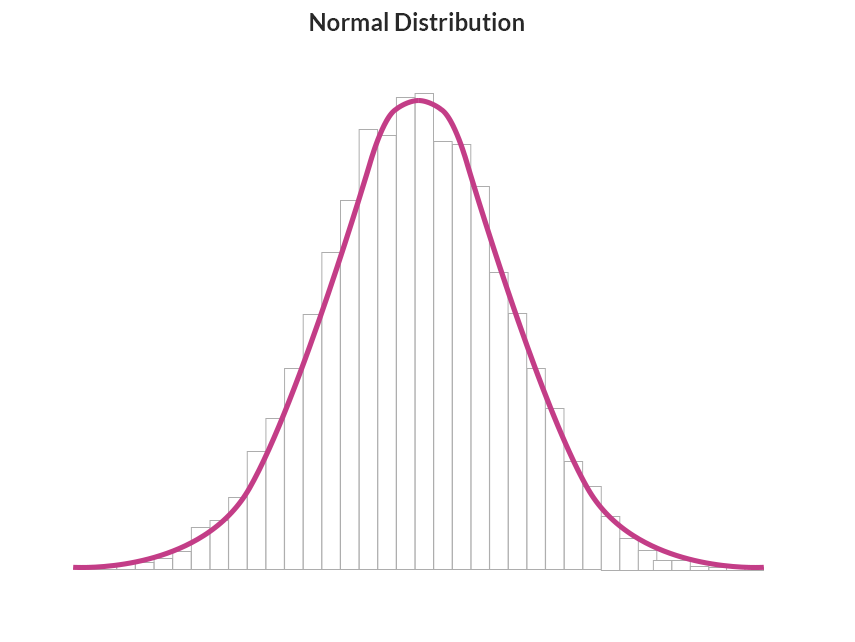In a standard normal distribution, the probability that a value lies more than couple of standard deviations away from the mean gets low very quickly. For example, just if you go just two standard deviations away from the mean, you cover around 95% of population; there is only around 5% of cases under the left and the right tail of the distribution curve.

### Skewness

Skewness is a parameter that describes asymmetry in a random variable's probability distribution. Skewness characterizes the degree of asymmetry of a distribution around its mean. Positive skewness indicates a distribution with an asymmetric tail extending toward more positive values. Negative skewness indicates a distribution with an asymmetric tail extending toward more negative values.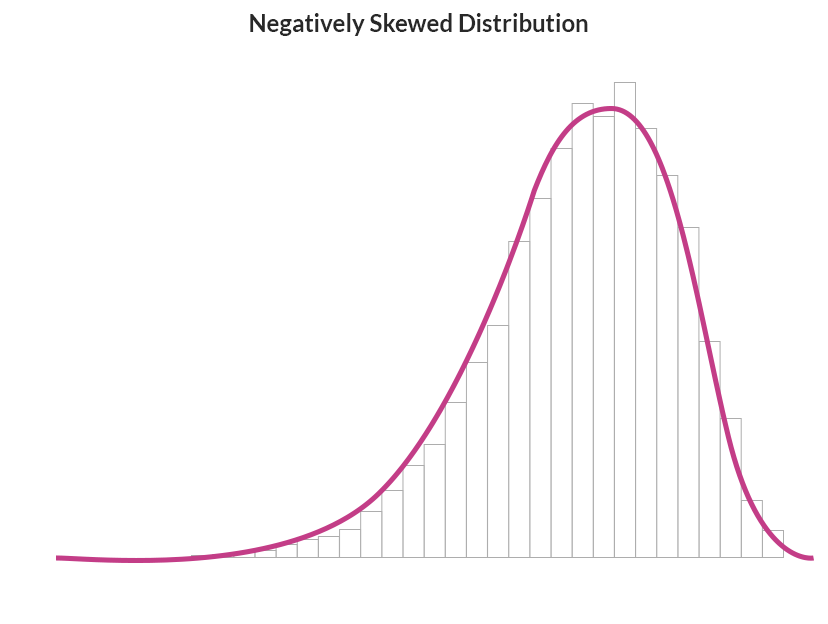Skewness tells you that values in the tail on one side of the mean (depending on whether the skewness is positive or negative) might still be valid, and you don't want to deal with them as outliers. Outliers are rare and far out-of-bounds values that might be erroneous. Therefore, knowing the skewness of a variable not only gives you only information about the variable distribution; it can also help you when you cleanse your data.

The formula for skewness is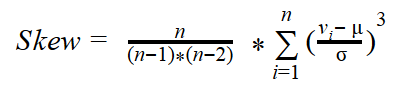The following figure shows a positively skewed distribution.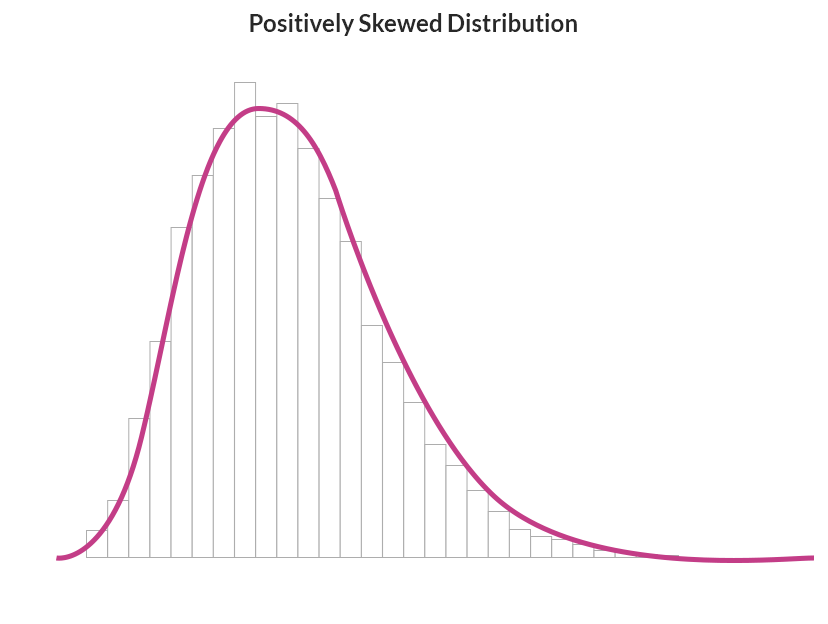#### Calculating the Skewness

The formula for the skewness uses the mean value and the standard deviation. In order to calculate those two values in advance, I would need to scan the data twice. I want to calculate the skewness by scanning the data only once.

I use a bit of mathematics for this optimization. First I expand the formula for the subtraction of the mean from the ith value on the 3rd degree: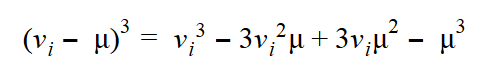Then I use the fact that the sum is distributive over the product, as shown in the formula for two values only: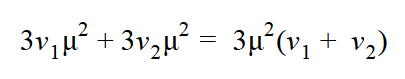This formula can be generalized for all values: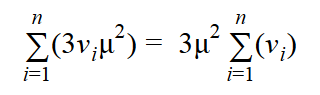I can do the same mathematics for the remaining elements of the expanded formula for the subtraction, calculating all the aggregates I need with a single pass through the data in a common table expression (CTE), and then calculate the skewness with these aggregates, as shown in the following query:

```USE AdventureWorksDW2014;
WITH SkewCTE AS
(
SELECT SUM(1.0*Age) AS rx,
SUM(POWER(1.0*Age,2)) AS rx2,
SUM(POWER(1.0*Age,3)) AS rx3,
COUNT(1.0*Age) AS rn,
STDEV(1.0*Age) AS stdv,
AVG(1.0*Age) AS av
FROM dbo.vTargetMail
)
SELECT
(rx3 - 3*rx2*av + 3*rx*av*av - rn*av*av*av)
/ (stdv*stdv*stdv) * rn / (rn-1) / (rn-2) AS Skewness
FROM SkewCTE;
```

The query returns the following result:

```Skewness
-----------------
0.708380928766786
```

Positive skewness means that the distribution of the Age variable has a longer tail on the right side, extending slightly more toward the positive values.

### Kurtosis

As mentioned, kurtosis characterizes the relative peakedness or flatness of a distribution compared with the normal distribution. A positive kurtosis means a higher peak around the mean and some extreme values on any side tail. A negative kurtosis indicates a relatively flat distribution. For a peaked distribution, consider that values far from the mean in any direction might be correct. The formula for the kurtosis is: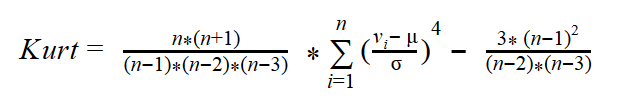The following figure shows a peaked distribution. Note that, compared to the standard distribution, it has more cases grouped around the mean value, but also longer tails on both sides.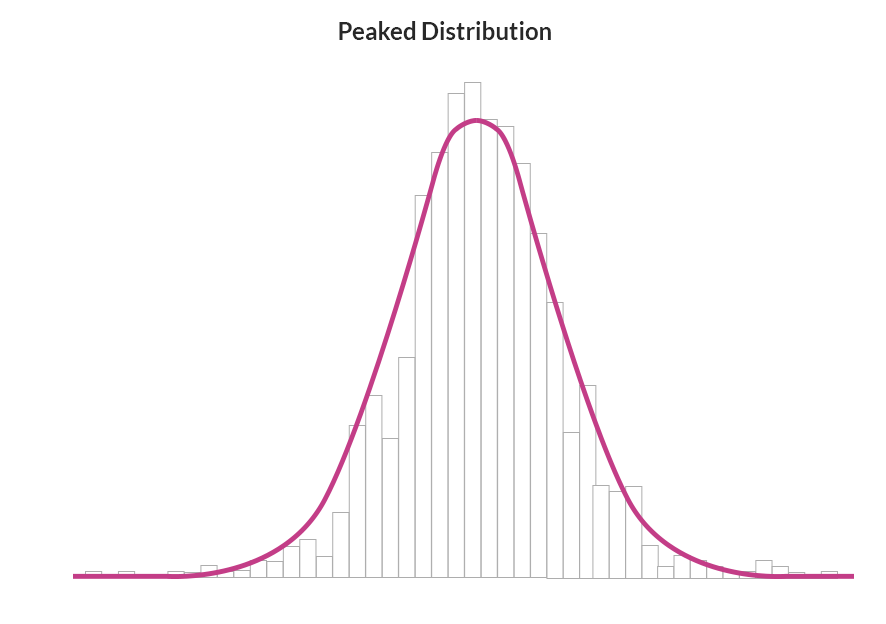#### Calculating the Kurtosis

As with the skewness, you can see that the formula for the kurtosis also includes the mean and the standard deviation. To get an efficient query, I start with expanding the subtraction again: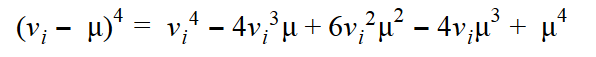After that, I can again use the fact that the sum is distributive over the product, and calculate the kurtosis with a single scan of the data, as the following query shows:

```WITH KurtCTE AS
(
SELECT SUM(1.0*Age) AS rx,
SUM(POWER(1.0*Age,2)) AS rx2,
SUM(POWER(1.0*Age,3)) AS rx3,
SUM(POWER(1.0*Age,4)) AS rx4,
COUNT(1.0*Age) AS rn,
STDEV(1.0*Age) AS stdv,
AVG(1.*Age) AS av
FROM dbo.vTargetMail
)
SELECT
(rx4 - 4*rx3*av + 6*rx2*av*av - 4*rx*av*av*av + rn*av*av*av*av)
/ (stdv*stdv*stdv*stdv) * rn * (rn+1) / (rn-1) / (rn-2) / (rn-3)
- 3.0 * (rn-1) * (rn-1) / (rn-2) / (rn-3) AS Kurtosis
FROM KurtCTE;
```

The result for the kurtosis of Age is:

```Kurtosis
-------------------
-0.0292548852625951
```

You can see that the distribution for Age is slightly flattened compared to the standard normal distribution.

### (*) For advanced: Skewness and kurtosis with CLR UDAs

The queries for calculating skewness and kurtosis are more complex than the queries earlier in the module. Now imagine you need to calculate these two values in groups. Calculating mean and standard deviation in groups is simple, because you have appropriate T-SQL aggregate functions which can be used together with the GROUP BY clause. However, calculating skewness and kurtosis in groups with T-SQL expressions leads only to more complex queries.

Calculating skewness and kurtosis in groups would be simple if the appropriate T-SQL aggregate functions existed. You can actually expand the list of the T-SQL aggregate functions with user-defined aggregate functions. However, you can't define a user-defined aggregate (UDA) in T-SQL. You need a Common Language Runtime (CLR) language for this—for example, Visual C#. You can use either an SQL Server Database Project template or a simple Class Library template in Microsoft Visual Studio, with the appropriate programming languages installed. Refer to books online for details on how to create such a project. Then you can simply copy the following C# code for the skewness and kurtosis UDAs, which uses the same mathematics as the T-SQL solutions for an efficient calculation with a single scan of the data.

Then you can build the project and later deploy the assembly (the .dll file) to your SQL Server instance. Of course you can also use the project with the source code — or the pre-built assembly provided with the accompanying code for the book.

#### Using the CLR UDAs

Once your assembly is built, you need to deploy it. The first step is to enable the CLR in your SQL Server instance.

```EXEC sp_configure 'clr enabled', 1;
RECONFIGURE WITH OVERRIDE;
```

Then you deploy the assembly. Deploying an assembly means importing it into a database. SQL Server does not rely on .dll files on disk. You can deploy an assembly from Visual Studio directly, or you can use the T-SQL CREATE ASSEMBLY command. The following command assumes that the DescriptiveStatistics.dll file exists in the C:\temp folder.

```CREATE ASSEMBLY DescriptiveStatistics
FROM 'C:\temp\DescriptiveStatistics.dll'
WITH PERMISSION_SET = SAFE;
```

After you import the assembly to your database, you can create the user-defined aggregates with the CREATE AGGREGATE command. The following two commands create the skewness and the kurtosis UDAs:

```-- Skewness UDA
CREATE AGGREGATE dbo.Skew(@s float)
RETURNS float
EXTERNAL NAME DescriptiveStatistics.Skew;
GO
-- Kurtosis UDA
CREATE AGGREGATE dbo.Kurt(@s float)
RETURNS float
EXTERNAL NAME DescriptiveStatistics.Kurt;
GO
```

After you deploy the UDAs, you can use them in the same way as you do standard T-SQL aggregate functions.

```SELECT dbo.Skew(1.0*Age) AS Skewness,
dbo.Kurt(1.0*Age) AS Kurtosis
FROM dbo.vTargetMail;
```

Now that you have the UDAs, of course, you can use them to calculate the skewness and the kurtosis in groups as well, as the following query shows.

```SELECT NumberCarsOwned,
AVG(1.0*Age) AS AgeMean,
STDEV(1.0*Age) AS AgeStandardDeviation,
dbo.Skew(1.0*Age) AS AgeSkewness,
dbo.Kurt(1.0*Age) AS AgeKurtosis
FROM dbo.vTargetMail
GROUP BY NumberCarsOwned
ORDER BY NumberCarsOwned;
```

### Conclusion

In this article you have learned again how important it is to know the mathematics behind the calculation. This knowledge helped me create a maximally efficient query. In addition, knowing how to write CLR aggregate functions and a CLR language like Visual C# is also very useful. With this knowledge I was able to write aggregate functions that extend the T-SQL built-in functions set.